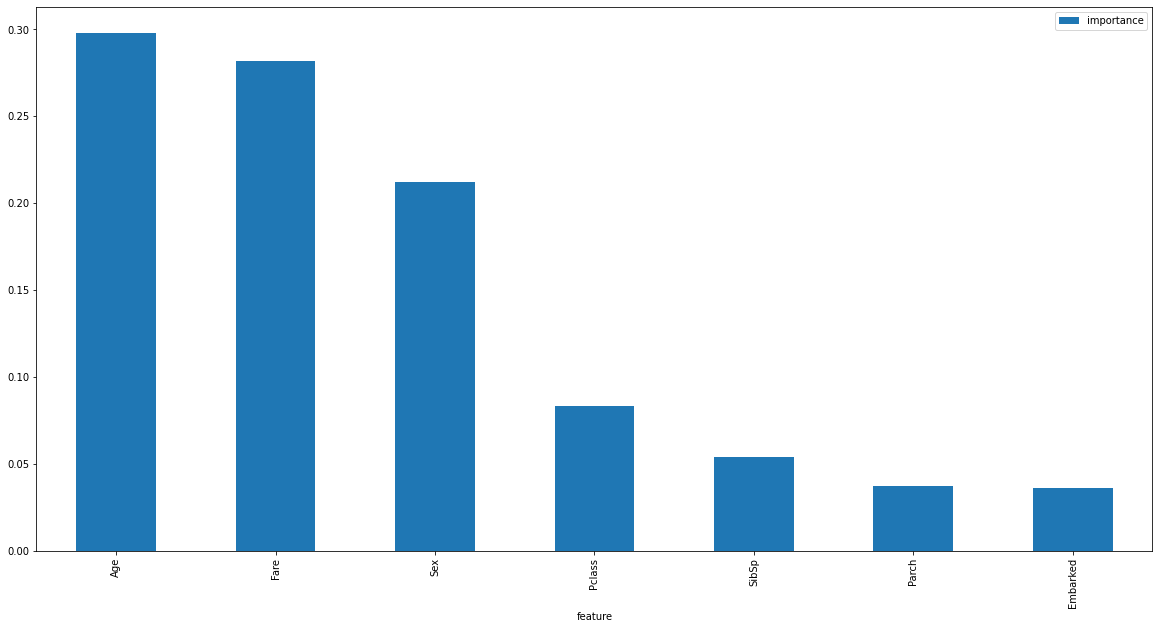# Titanic EDA (Exploratory Data Analysis)¶

Compiled by Karl Duckett - March 2021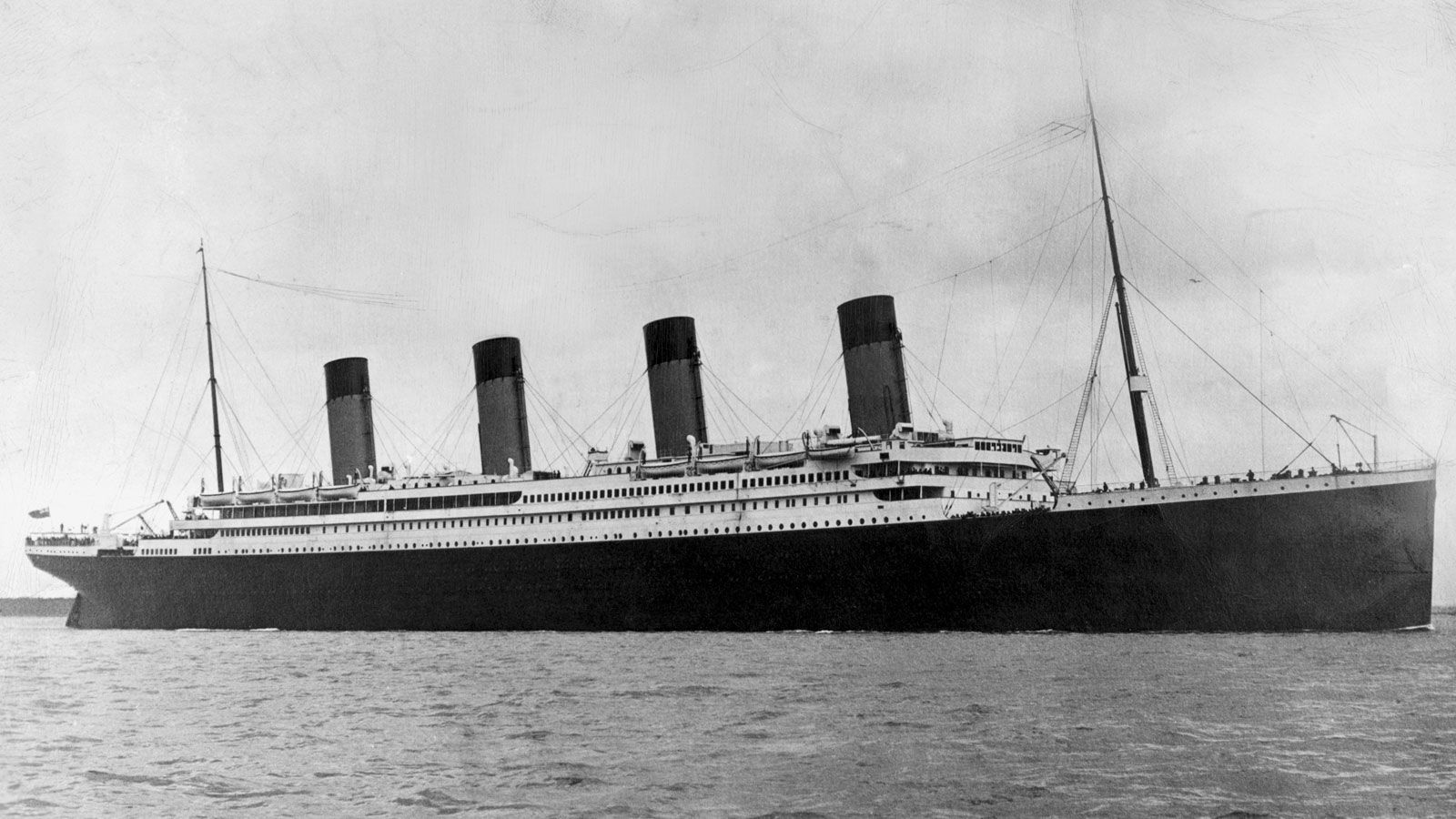Image source Britannica.com

RMS Titanic was a British passenger liner operated by the White Star Line that sank in the North Atlantic Ocean on 15 April 1912, after striking an iceberg during her maiden voyage from Southampton to New York City. Of the estimated 2,224 passengers and crew aboard, more than 1,500 died, making the sinking at the time one of the deadliest of a single ship and the deadliest peacetime sinking of a superliner or cruise ship to date. With much public attention in the aftermath the disaster has since been the material of many artistic works and a founding material of the disaster film genre.

In :
import numpy as np
import pandas as pd
import seaborn as sns
import matplotlib.pyplot as plt
import ipy_table as tbl
from numbers import Number
from scipy import stats

plt.rcParams['figure.figsize'] = (20, 10)

In :
titanic = pd.read_csv('titanic.csv')

In :
titanic['class'] = titanic['Pclass'].replace({3 : 'third', 2 : 'second', 1:'first'})
titanic['gender'] = titanic['Sex'].replace({'male' : 'man', 'female':'woman'})
titanic['port'] = titanic['Embarked'].replace({'Q':'Queenstown', 'C':'Cherbourg', 'S':'Southampton'})

In :
def get_who(titanic):
if(titanic['Age'] < 18):
return 'child'
else:
return titanic['gender']

In :
titanic['who'] = titanic.apply(get_who, axis = 1)

In :
titanic.head()

Out:
PassengerId Survived Pclass Name Sex Age SibSp Parch Ticket Fare Cabin Embarked class gender port alive who
0 1 0 3 Braund, Mr. Owen Harris male 22.0 1 0 A/5 21171 7.2500 NaN S third man Southampton dead man
1 2 1 1 Cumings, Mrs. John Bradley (Florence Briggs Th... female 38.0 1 0 PC 17599 71.2833 C85 C first woman Cherbourg alive woman
2 3 1 3 Heikkinen, Miss. Laina female 26.0 0 0 STON/O2. 3101282 7.9250 NaN S third woman Southampton alive woman
3 4 1 1 Futrelle, Mrs. Jacques Heath (Lily May Peel) female 35.0 1 0 113803 53.1000 C123 S first woman Southampton alive woman
4 5 0 3 Allen, Mr. William Henry male 35.0 0 0 373450 8.0500 NaN S third man Southampton dead man
In :
# Count the number of rows and columns in the dataset
titanic.shape

Out:
(891, 17)
In :
titanic.describe()

Out:
PassengerId Survived Pclass Age SibSp Parch Fare
count 891.000000 891.000000 891.000000 714.000000 891.000000 891.000000 891.000000
mean 446.000000 0.383838 2.308642 29.699118 0.523008 0.381594 32.204208
std 257.353842 0.486592 0.836071 14.526497 1.102743 0.806057 49.693429
min 1.000000 0.000000 1.000000 0.420000 0.000000 0.000000 0.000000
25% 223.500000 0.000000 2.000000 20.125000 0.000000 0.000000 7.910400
50% 446.000000 0.000000 3.000000 28.000000 0.000000 0.000000 14.454200
75% 668.500000 1.000000 3.000000 38.000000 1.000000 0.000000 31.000000
max 891.000000 1.000000 3.000000 80.000000 8.000000 6.000000 512.329200
In :
# Get a count of the number of survivors
titanic['alive'].value_counts()

Out:
dead     549
alive    342
Name: alive, dtype: int64
In :
# Visualize the count of survivors
sns.countplot(x = titanic['alive']);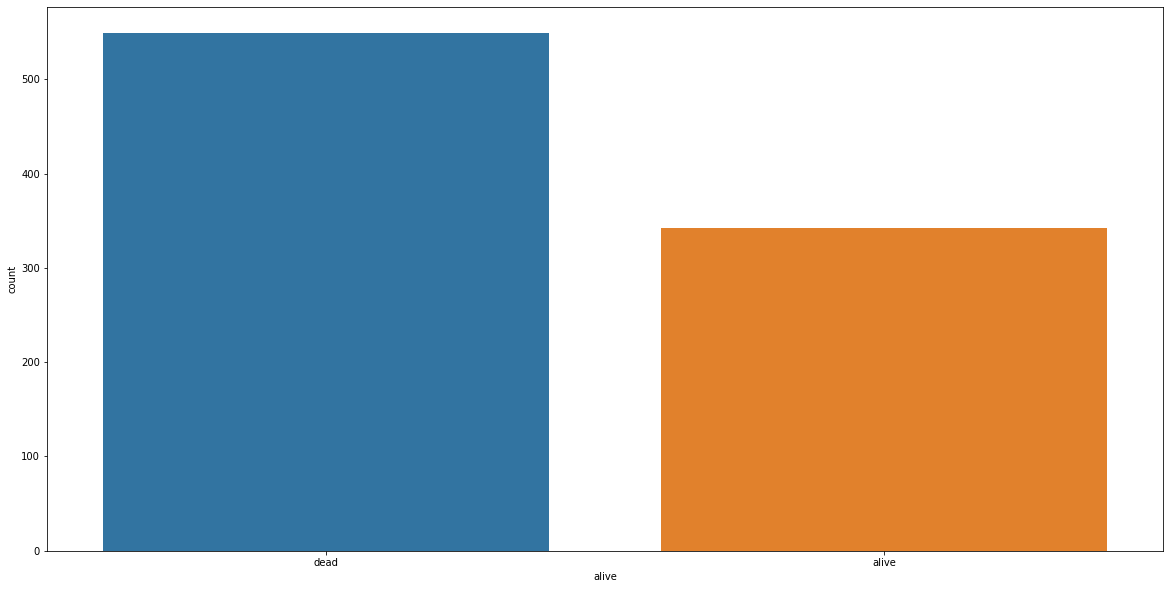In :
cols = ['who', 'Sex', 'Pclass', 'SibSp', 'Parch', 'Embarked']
n_rows = 2
n_cols = 3

# The subplot grid and figure size of each graph
fig, axs = plt.subplots(n_rows, n_cols, figsize = (n_cols * 6.2, n_rows * 6.2))

for r in range(0, n_rows):
for c in range(0, n_cols):
i = r * n_cols + c # Creating the index to go through the number of columns
ax = axs[r][c] # Show where to position each sub plot
sns.countplot(x = titanic[cols[i]], hue=titanic['alive'], ax=ax) # Create the plot for each columns in col array
ax.set_title(cols[i])
ax.legend(title = 'alive', loc = 'upper right')

plt.tight_layout()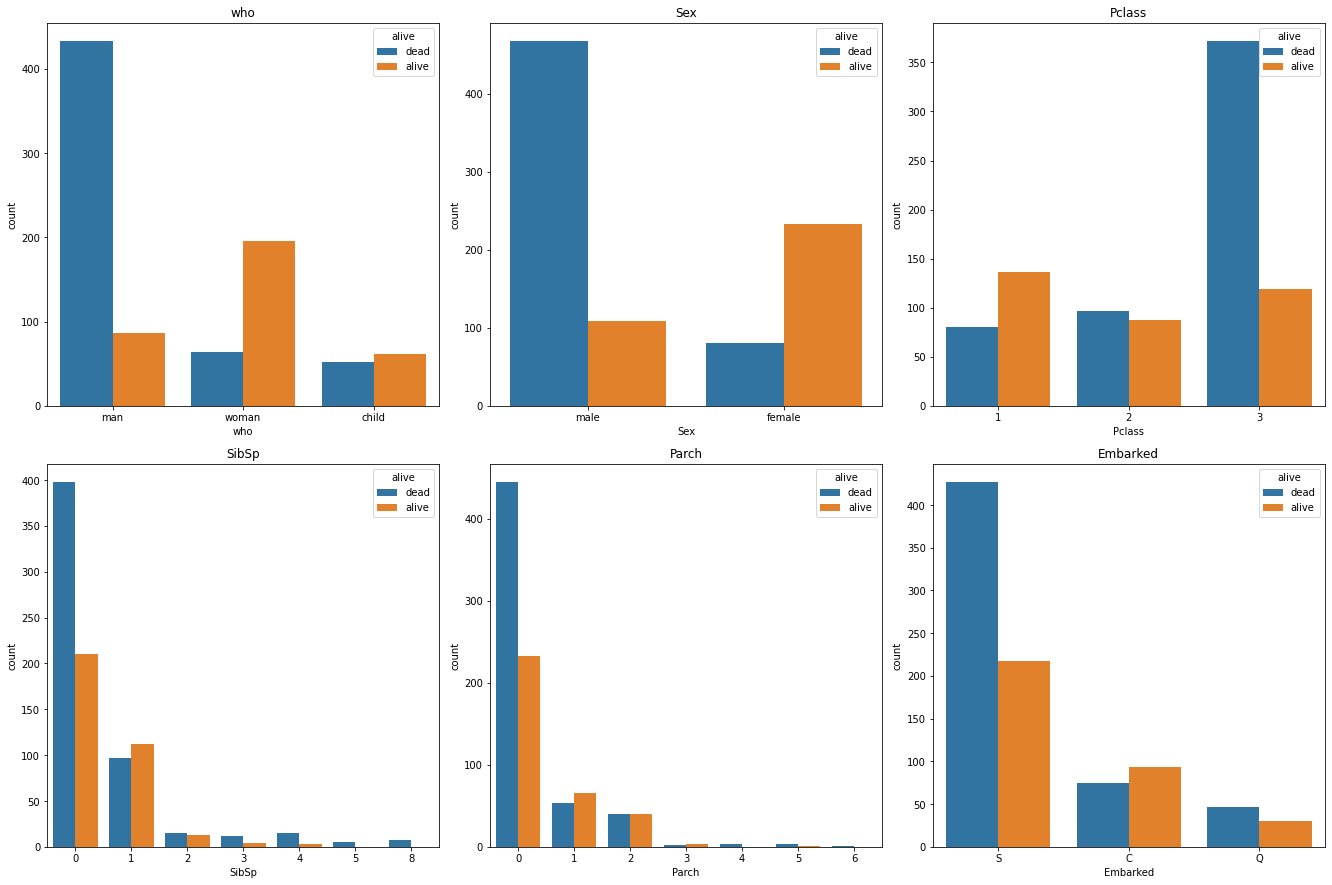The charts above show us that men, males, third class, travelling alone had the worst survival rate.

In :
# check the ages of the passengers
# quick way to get the histogram is using pandas itself
titanic['Age'].hist(bins=70);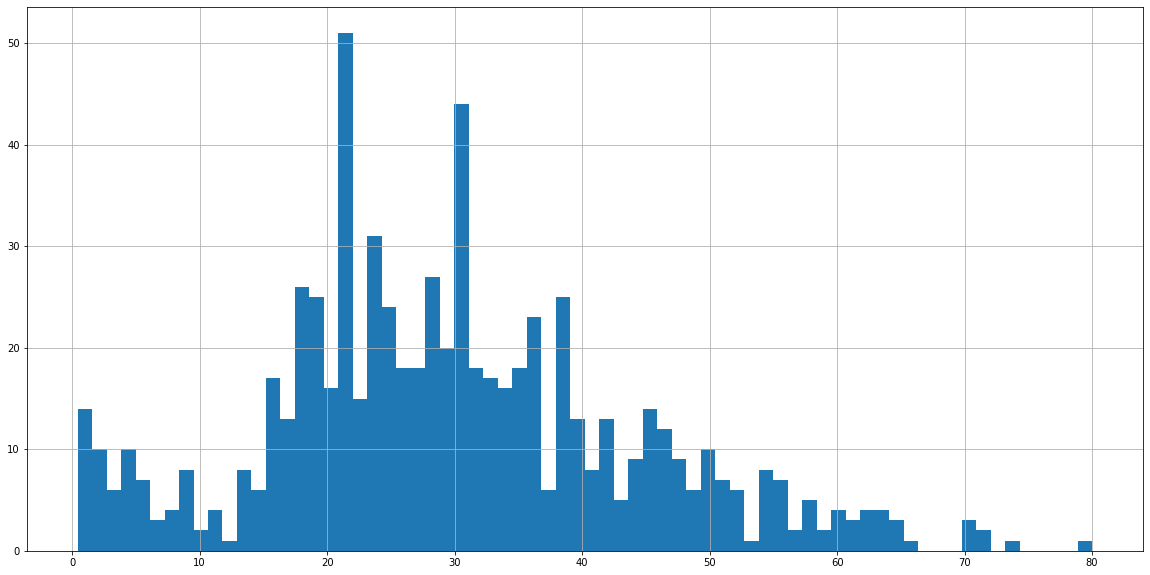In :
# Another value to visualize the data is to use FacetGrid to plot multiple kdeplots on one plot
#Set the figure equal to a facetgrid with the pandas dataframe as its source, set the hue, and aspect ratio.

fig = sns.FacetGrid(titanic, hue='Sex', aspect=4)

#next use map to plot all the possible kdeplots for the ‘Age’ column by the hue choice

# setting the upper limit by the oldest passenger
oldest = titanic['Age'].max()

# since we know that no one can be of negative age, we will set the lowest limit as zero
fig.set(xlim=(0, oldest))
plt.gcf().set_size_inches(17.7, 6.27)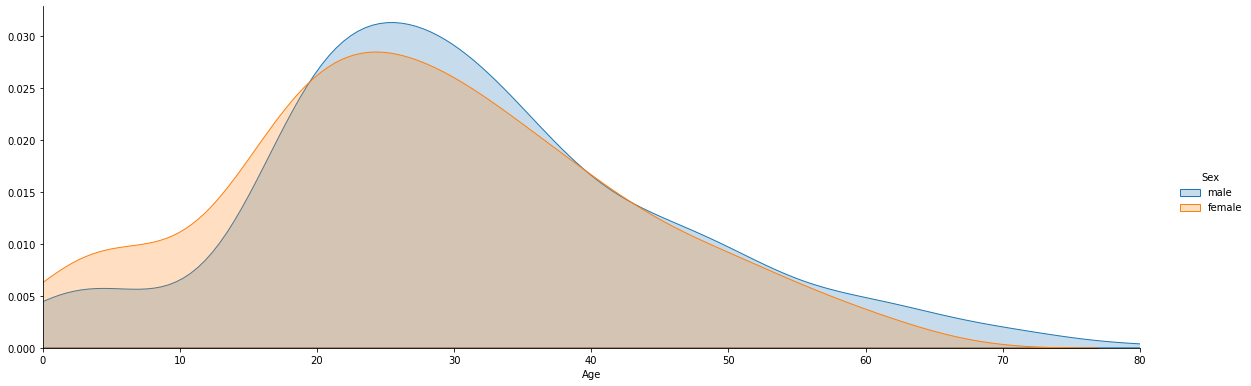Let's do a normal test on the age distribution

H0: The data follows the normal distribution

H1: The data do not follow the normal distribution

In :
import scipy.stats as sc
import statsmodels.graphics.gofplots as sm

data = titanic['Age']

standard_norm = data

# plots for standard distribution
sns.histplot(standard_norm,kde=True, color ='blue')
sm.ProbPlot(standard_norm).qqplot(line='s');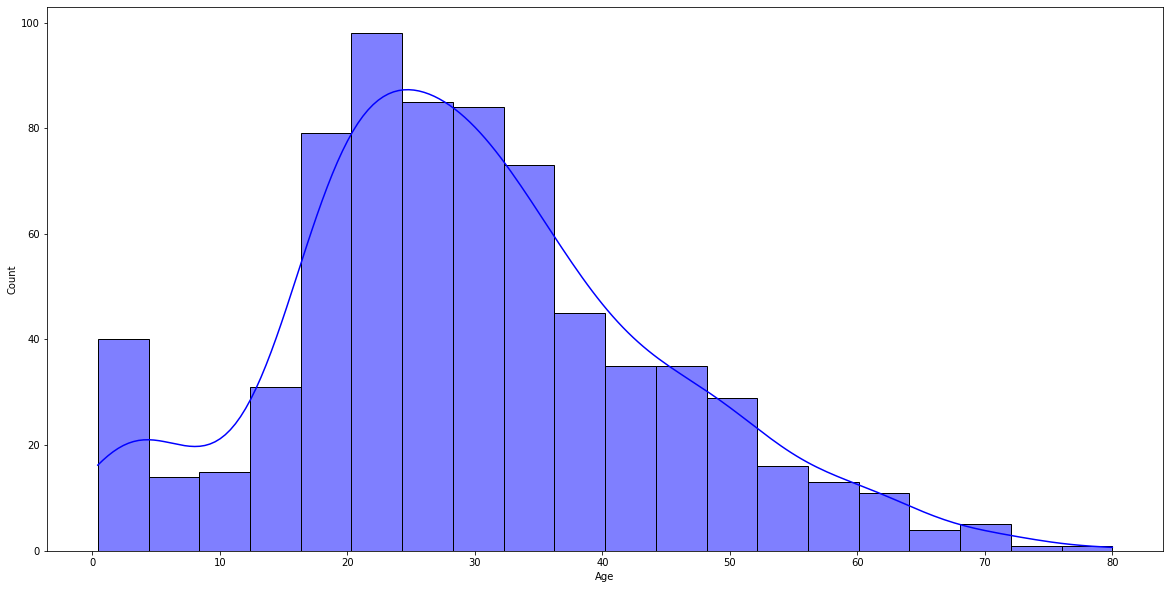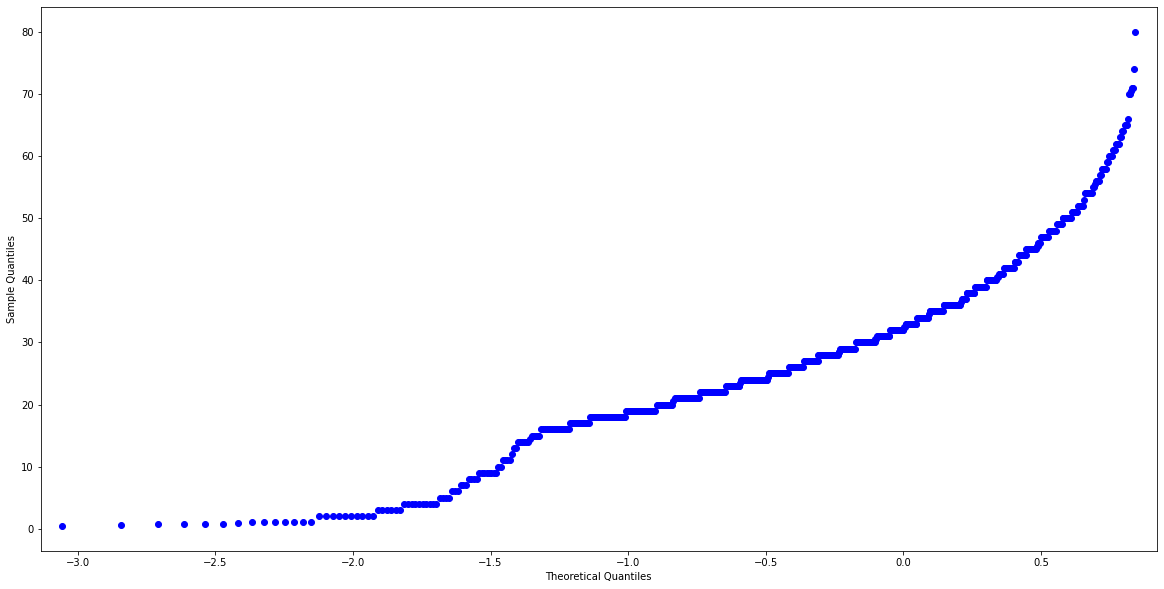In :
from scipy.stats import anderson

titanic_no_na_age = titanic.dropna(subset=['Age'])
data = titanic_no_na_age['Age']
results = anderson(data)
print(results)

AndersonResult(statistic=3.8230373853616584, critical_values=array([0.573, 0.652, 0.783, 0.913, 1.086]), significance_level=array([15. , 10. ,  5. ,  2.5,  1. ]))

In :
titanic.groupby('Sex')[['Survived']].mean()

Out:
Survived
Sex
female 0.742038
male 0.188908
In :
# Look at the survival rate by sex and class
titanic.pivot_table('Survived', index = 'who', columns = 'class')

Out:
class first second third
who
child 0.916667 0.913043 0.371795
man 0.347458 0.082474 0.121711
woman 0.976744 0.906250 0.486239
In :
# Look at the survival rate by sex and class visually
titanic.pivot_table('Survived', index = 'who', columns = 'class').plot();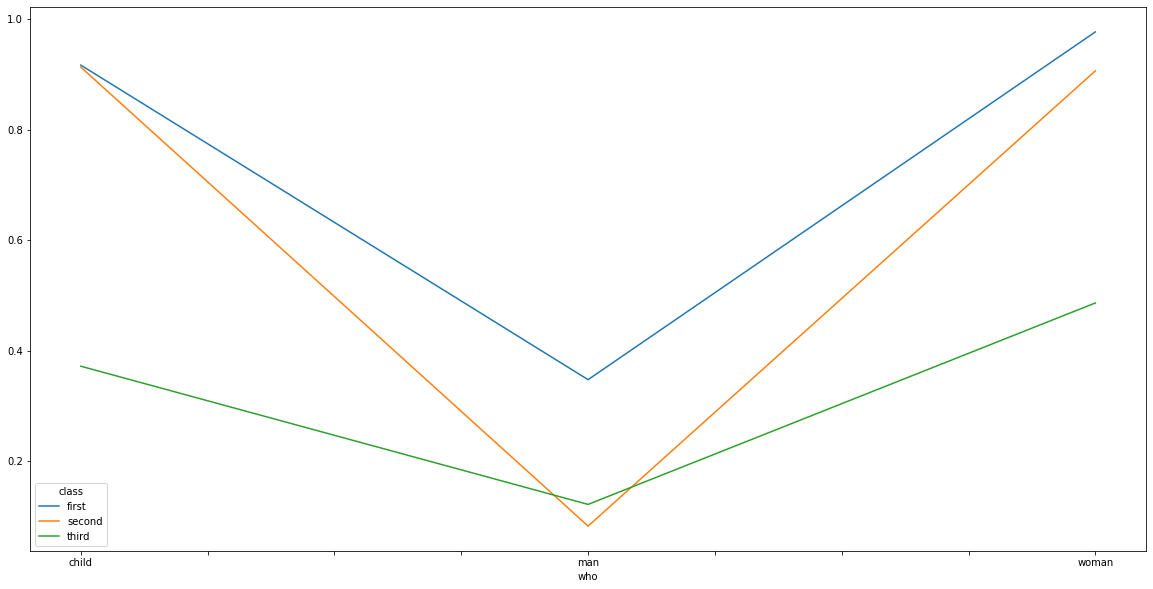In :
# Plot the suvival rate of each class
sns.barplot(x = 'Pclass', y = 'Survived', data = titanic);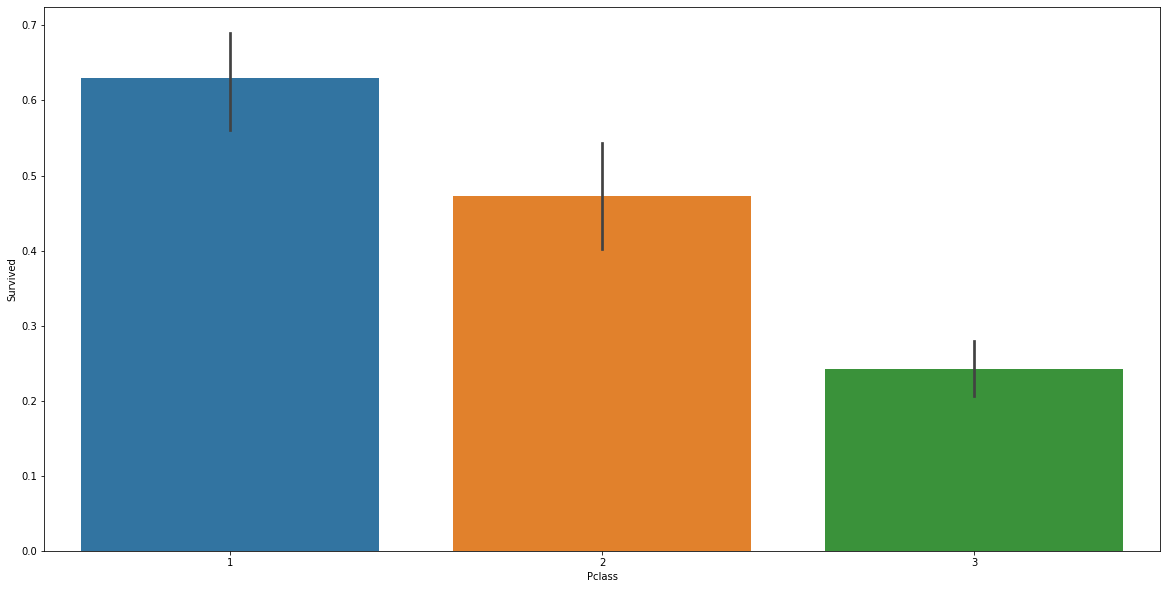In :
# Look at survival rate by sex, age and class
age = pd.cut(titanic['Age'], [0, 18, 80])
titanic.pivot_table('Survived', ['Sex', age], 'class')

Out:
class first second third
Sex Age
female (0, 18] 0.909091 1.000000 0.511628
(18, 80] 0.972973 0.900000 0.423729
male (0, 18] 0.800000 0.600000 0.215686
(18, 80] 0.375000 0.071429 0.133663
In :
age = pd.cut(titanic['Age'], [0, 18, 80])
titanic.pivot_table('Survived', ['Sex', age], 'class').plot();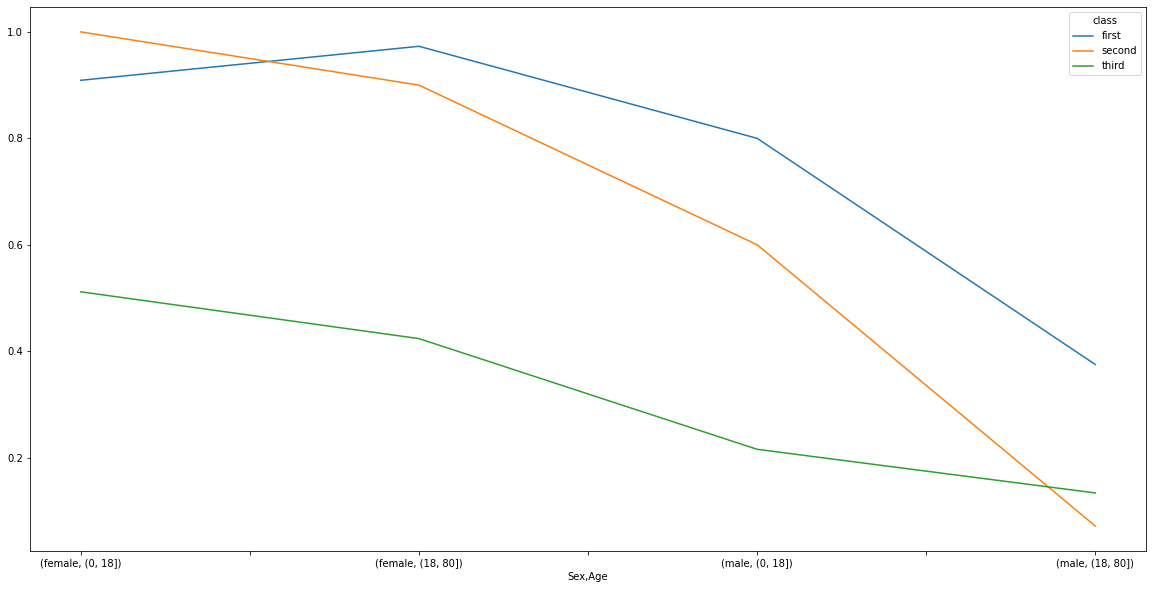In :
order = ['first', 'second', 'third'].reverse()
titanic['class'] = pd.Categorical(titanic['class'], order)
titanic.sort_values(by=['class'], inplace=True)

plt.scatter(x = titanic['Fare'], y =  titanic['class'], color = 'purple', label = 'Passenger Paid')
plt.ylabel("Class")
plt.xlabel("Price / Fare")
plt.title("Price of each class")
plt.legend()
plt.show();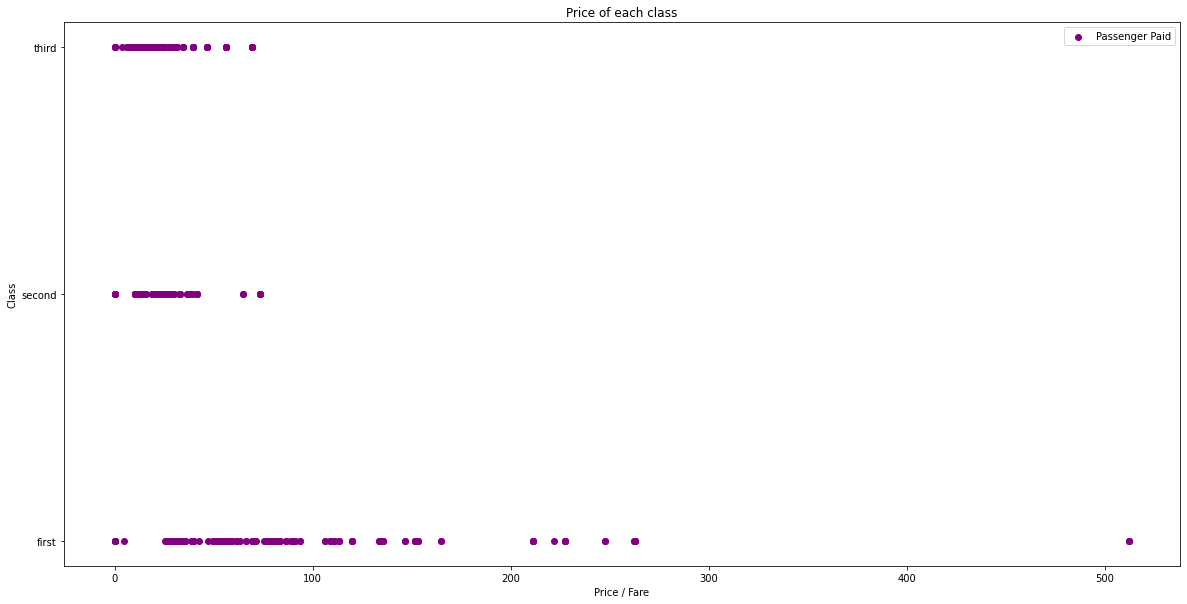Looking at the names of the passengers there are some interesting titles of passengers. Let's have a look at the survival counts of each of these titles of passengers.

In :
def extract_title(name):
names = list(name.split(' '))
return names

In :
titanic['title'] = titanic['Name'].apply(extract_title)

In :
titanic.pivot_table('Survived','title', 'alive',aggfunc='count').fillna(0).astype(int)

Out:
title
Billiard, 0 1
Capt. 0 1
Carlo, 0 1
Col. 1 1
Cruyssen, 0 1
Don. 0 1
Dr. 3 4
Gordon, 2 0
Impe, 0 3
Jonkheer. 0 1
Major. 1 1
Master. 23 17
Melkebeke, 0 1
Messemaeker, 1 0
Miss. 126 53
Mlle. 2 0
Mme. 1 0
Mr. 79 423
Mrs. 97 24
Ms. 1 0
Mulder, 1 0
Pelsmaeker, 0 1
Planke, 0 3
Rev. 0 6
Shawah, 0 1
Steen, 0 1
Velde, 0 1
Walle, 0 1
der 0 1
the 1 0
y 3 1

## Which variables are the largest factors in the chance of surviving the Titanic disaster?¶

In :
# Count the empty values in each column
titanic.isna().sum()

Out:
PassengerId      0
Survived         0
Pclass           0
Name             0
Sex              0
Age            177
SibSp            0
Parch            0
Ticket           0
Fare             0
Cabin          687
Embarked         2
class            0
gender           0
port             2
alive            0
who              0
title            0
dtype: int64
In :
# We can visualise a sample of the null values in each column with a heatmap
plt.figure(figsize=(17,5))
sns.heatmap(titanic.isnull(), cbar=False);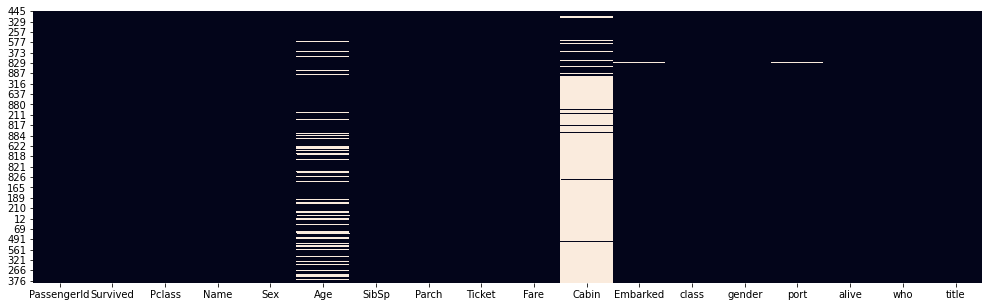In :
# Remove the following columns (by name)
titanic = titanic.drop(['PassengerId', 'Name', 'Ticket', 'gender', 'port', 'Cabin', 'who', 'alive', 'class'], axis = 1)

In :
# Remove the rows with missing values (column specific using subset)
titanic = titanic.dropna(subset=['Embarked','Age'])

In :
# Count the NEW number of rows and columns in the dataset
titanic.shape

Out:
(712, 9)
In :
# Let's look at the data types
titanic.dtypes

Out:
Survived      int64
Pclass        int64
Sex          object
Age         float64
SibSp         int64
Parch         int64
Fare        float64
Embarked     object
title        object
dtype: object
In :
print(titanic['Sex'].unique())
print(titanic['Embarked'].unique())

['male' 'female']
['S' 'C' 'Q']

In :
# Let's convert the non int or float values to numerical columns
from sklearn.preprocessing import LabelEncoder

In :
labelencoder = LabelEncoder()

In :
titanic.iloc[:,2] = labelencoder.fit_transform(titanic.iloc[:,2].values)
titanic.iloc[:,7] = labelencoder.fit_transform(titanic.iloc[:,7].values)
print(titanic['Sex'].unique())
print(titanic['Embarked'].unique())

[1 0]
[2 0 1]

In :
titanic.dtypes

Out:
Survived      int64
Pclass        int64
Sex           int32
Age         float64
SibSp         int64
Parch         int64
Fare        float64
Embarked      int32
title        object
dtype: object
In :
# Split the data into independent 'X' and dependent 'Y' variables
X = titanic.iloc[:, 1:8].values     # Note starting at column 1 as column 0 is the survived (we will be using that to predict)
Y = titanic.iloc[:, 0].values

In :
# Split the dataset into 80% training and 20% testing
from sklearn.model_selection import train_test_split
X_train, X_test, Y_train, Y_test = train_test_split(X, Y, test_size = 0.2, random_state = 0)

In :
# Scale the data
from sklearn.preprocessing import StandardScaler
sc = StandardScaler()
X_train = sc.fit_transform(X_train)
X_test = sc.fit_transform(X_test)

In :
# Create a function wtih many machine leanring models
def models(X_train, Y_train):
# Use logistic regression
from sklearn.linear_model import LogisticRegression
log = LogisticRegression(random_state = 0)
log.fit(X_train, Y_train)

# Use K-nearest neighbours
from sklearn.neighbors import KNeighborsClassifier
knn = KNeighborsClassifier(n_neighbors = 5, metric = 'minkowski', p = 2)
knn.fit(X_train, Y_train)

# Use Support Vector Classifiers (linear kernel)
from sklearn.svm import SVC
svc_lin = SVC(kernel='linear', random_state = 0)
svc_lin.fit(X_train, Y_train)

# Use Support Vector Classifier (RBF Kernel)
from sklearn.svm import SVC
svc_rbf = SVC(kernel='rbf', random_state = 0)
svc_rbf.fit(X_train, Y_train)

# Use GaussianNB
from sklearn.naive_bayes import GaussianNB
gauss = GaussianNB()
gauss.fit(X_train, Y_train)

# Use Decision tree classifier
from sklearn.tree import DecisionTreeClassifier
tree = DecisionTreeClassifier(criterion = 'entropy', random_state = 0)
tree.fit(X_train, Y_train)

# Use Random Forest Classifier
from sklearn.ensemble import RandomForestClassifier
forest = RandomForestClassifier(n_estimators=10, criterion = 'entropy', random_state = 0)
forest.fit(X_train, Y_train)

# Print the training accuracy for each model tested
print(" Logistic Regression Training Accuracy: ", log.score(X_train, Y_train))
print(" K Neighbords Training Accuracy: ", knn.score(X_train, Y_train))
print(" SVC Linear Training Accuracy: ", svc_lin.score(X_train, Y_train))
print(" SVC RBF Training Accuracy: ", svc_rbf.score(X_train, Y_train))
print(" Gaussian Training Accuracy: ", gauss.score(X_train, Y_train))
print(" Decision Tree Training Accuracy: ", tree.score(X_train, Y_train))
print(" Random Forest Training Accuracy: ", forest.score(X_train, Y_train))

return log, knn, svc_lin, svc_rbf, gauss, tree, forest

In :
# Get and train all of the models
model = models(X_train, Y_train)

 Logistic Regression Training Accuracy:  0.8084358523725835
 K Neighbords Training Accuracy:  0.875219683655536
 SVC Linear Training Accuracy:  0.7750439367311072
 SVC RBF Training Accuracy:  0.843585237258348
 Gaussian Training Accuracy:  0.7908611599297012
 Decision Tree Training Accuracy:  0.9876977152899824
 Random Forest Training Accuracy:  0.9718804920913884

In :
# Show the confusion matrix and accuracy for all of the models on the test data (20%)
from sklearn.metrics import confusion_matrix

for i in range(len(model)):
cm = confusion_matrix(Y_test, model[i].predict(X_test))
# Extract the True Negative, False Positive, False Negative and the True Positive
TN, FP, FN, TP = confusion_matrix(Y_test, model[i].predict(X_test)).ravel()

test_score = (TP + TN)  / (TP + TN + FN + FP)

print(cm)
print('Model [{}] Testing Accuracy = "{}"'.format(i, test_score))
print()

[[70 14]
[15 44]]
Model  Testing Accuracy = "0.7972027972027972"

[[71 13]
[17 42]]
Model  Testing Accuracy = "0.7902097902097902"

[[71 13]
[16 43]]
Model  Testing Accuracy = "0.7972027972027972"

[[78  6]
[18 41]]
Model  Testing Accuracy = "0.8321678321678322"

[[74 10]
[21 38]]
Model  Testing Accuracy = "0.7832167832167832"

[[70 14]
[19 40]]
Model  Testing Accuracy = "0.7692307692307693"

[[76  8]
[16 43]]
Model  Testing Accuracy = "0.8321678321678322"


In :
# Get feature importance
forest = model # Using the most successful model - random forest classifier
importances = pd.DataFrame({'feature' : titanic.iloc[:, 1:8].columns, 'importance' : np.round(forest.feature_importances_, 3)})
importances = importances.sort_values('importance', ascending = False).set_index('feature')
importances

Out:
importance
feature
Age 0.298
Fare 0.282
Sex 0.212
Pclass 0.083
SibSp 0.054
Parch 0.037
Embarked 0.036
In :
# Visualize the importance
importances.plot.bar();